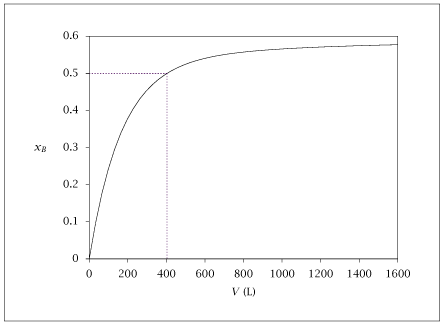## Figure 4.20:

### Benzene conversion versus reactor volume.## Code for Figure 4.20

### main.m

```
1
2
3
4
5
6
7
8
9
10
11
12
13
14
15
16
17
18
19
20
21
22
23
24
25
26
27
28
29
30
31
32
33
34
35
36
37
38
39
40
41
42
43
44
45
46
47
48
49
50
51
52
53
54
55
56
57
58
59
60
61
62
63
64
65
66
67
68
69
70% Copyright (C) 2001, James B. Rawlings and John G. Ekerdt
%
% This program is free software; you can redistribute it and/or
% modify it under the terms of the GNU General Public License as
% published by the Free Software Foundation; either version 2, or (at
% your option) any later version.
%
% This program is distributed in the hope that it will be useful, but
% WITHOUT ANY WARRANTY; without even the implied warranty of
% MERCHANTABILITY or FITNESS FOR A PARTICULAR PURPOSE.  See the GNU
% General Public License for more details.
%
% You should have received a copy of the GNU General Public License
% along with this program; see the file COPYING.  If not, write to
% the Free Software Foundation, 59 Temple Place - Suite 330, Boston,
% MA 02111-1307, USA.

% Revised 7/24/2018

% Example in material balance chapter.
% Data are:
%           NBf = 60e3 mol/hr
%           R  = 0.08205 L*atm/mol K
%           T  = 1033 K
%           P  = 1 atm
%           k1 = 7e5 L/mol hr
%           k2 = 4e5 L/mol hr
%           K1 = 0.31
%           K2 = 0.48
%           Reactor volume: 0 to 1600 L

p = struct();
p.NBf = 60e3;
p.R  = 0.08205;
p.T  = 1033;
p.P  = 1;
p.k1 = 7e5;
p.k2 = 4e5;
p.K1 = 0.31;
p.K2 = 0.48;

x0=[0;0];

vout = linspace(0,1600,50)';

opts = odeset ('AbsTol', sqrt (eps), 'RelTol', sqrt (eps));
[tsolver, x] = ode15s(@(vol,x) benzene_pyrol_rhs(vol,x,p), vout, x0, opts);

conv = ( 2*x(:,1) + x(:,2) )/p.NBf;
yB   = ( p.NBf - 2*x(:,1) - x(:,2) )/p.NBf;
yD   = ( x(:,1) - x(:,2) )/p.NBf;
yT   = ( x(:,2) )/p.NBf;
yH   = ( x(:,1) + x(:,2) )/p.NBf;

data = [vout conv yB yD yT yH];
aux_data = [403.25 .50  403.25 .50; 403.25 0.0  0.0 .50];
save benz_pyrol.dat data aux_data;

if (~ strcmp (getenv ('OMIT_PLOTS'), 'true')) % PLOTTING
subplot(2,1,1);
plot (data(:,1),data(:,2));
axis([0 1600 0 .6])
% TITLE

subplot(2,1,2);
plot (data(:,1),data(:,3:6));
axis([0 1600 0 1])
% TITLE
end % PLOTTING

```

### benzene_pyrol_rhs.m

```
1
2
3
4
5
6
7
8
9
10
11
12
13
14
15
16
17
18function xdot = benzene_pyrol_rhs(volume,x,p)
ext1 = x(1);
ext2 = x(2);

NB = p.NBf - 2*ext1 - ext2;
ND = ext1 - ext2;
NH = ext1 + ext2;
NT = ext2;

Q = p.NBf*p.R*p.T/p.P;

cB = NB/Q;
cD = ND/Q;
cT = NT/Q;
cH = NH/Q;

xdot = [p.k1*(cB*cB - cD*cH/p.K1);
p.k2*(cB*cD - cT*cH/p.K2)];

```